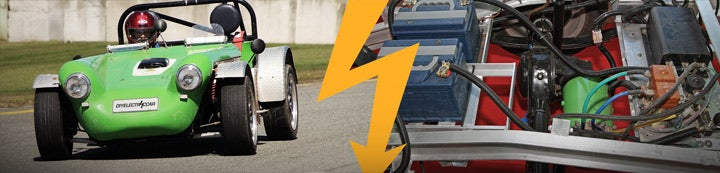1 - 3 of 3 Posts

#### kennybobby

·
##### Registered
Joined
·
1,442 Posts
Discussion Starter
One thing that we have found is that the chargers send out a long serial stream of data (79 bytes) that is updated about every 2 seconds. One surprise was that the chargers send out the number of Amp-hr that have been put into the battery pack during the charge cycle. This is a floating point number that is sent out as 4 bytes of hex code.

The following spreadsheet has a formula for converting 4 bytes of hex to the decimal number.

The formula in the cell that does the calculation is this:
=((-1)^LEFT(HEX2BIN(A3,8),1))*(2^(BIN2DEC(CONCATENATE(RIGHT(HEX2BIN(A3,8),7),LEFT(HEX2BIN(A4,8),1)))-127))*(1+(BIN2DEC(RIGHT(HEX2BIN(A4,8),7))/128)+(HEX2DEC(A5)/(2*128^2))+(HEX2DEC(A6)/(4*128^3)))

Another way to convert is to use an online calc u later such as found here:
http://www.h-schmidt.net/FloatConverter/IEEE754.html

The details on how to extract this data is in work and will be posted asap. This could be used as a 'fuel gauge' to show fill-up of the pack.

#### Attachments

• 24.5 KB Views: 15

#### z_power

·
##### Registered
Joined
·
220 Posts
Hi kb,
Would you mind sharing your findings on this 79 bytes long frames? I'm green on reverse engineering protocols, tried to decipher it today - only found length and divided it into few blocks. I'm a bit stuck at the moment because only observations I could take were on charger idliing (need a few days to finish battery box and mount cells inside) but I'd gladely contribute to this project.
Mike

#### piotrsko

·
##### Registered
Joined
·
1,548 Posts
Did you ever determine how to talk to the controllers? I saw some mention of RST commands.

1 - 3 of 3 Posts